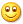# 2018年第十一 周 周二 大盘预测

[2018-03-13 07:09:48]

2018年第十一   周二  大盘预测

【中证500

K线标值：MACD (-1) KDJ443464 SAR （红）=5766

13日量  12253万手 55日量 12121万手

线序 13日线6005  43日线 6092 120日线6294  250日线6220

W&R=32 RSI(2,3)=6450   B5 =1 ARBR（）= 109 98），CCI= 11

===========

【上证】

K线标值：MACD (-47)  KDJ434930  SAR （红）=3233

13日量16476 万手  55日量 18980万手

线序 13日线3285  43日线 3373 120日线3361  250日线3282  909日线 3192

W&R=49 RSI(2,3)=5349   B5 =0 ARBR（）= 117 81），CCI=-27

================

【中小板】

K线图：MACD ( 20)  KDJ493675  SAR （红）=7192

13日量  7214万手 55日量 6726万手

线序 13日线7498  43日线 7466 120日线7611  250日线7205   909日线7019

W&R=26 RSI(2,3)=6553   B5 = 3 ARBR（）= 117 91），CCI= 59

====================

【创业板】

K线图：MACD ( 35)  KDJ553496  SAR （红）=1695

13日量5079 万手  55日量3973 万手

W&R=8 RSI(2,3)=7253 B5 =7 ARBR（）= 160 116），CCI= 147

【中证500

60分钟：展：强势 R(3)=55  弱势上影线阴落。R(3)=53-

15分钟强势顶冲，见D=95 R(3)=65  弱势 下破13线，R(3)=59-

【上证】

60分钟：展：强势立上120线和250线。 弱势下落13线。

15分钟 强势顶冲R(3)=59 909线下压、 弱势下破13线，

【中小板】

60分钟：  展：强势顶冲R(3)=60   弱势

15分钟强势顶冲D=90 R(3)=69  弱势下破13线，R(3)=60-

【创业板】

60分钟：展：强势顶冲R(3)=60   弱势上影线下落13线。

15分钟强势顶冲D=90 R(3)=69  弱势 下破13线，R(3)=59-

【中证5005分钟强势 阳升新高，R(3)=65   弱势 下破34线，R(3)=59-

【上证】5分钟 强势顶冲R(3)=60  弱势下落R(3)=55-

【中小】5分钟 强势顶冲R(3)=65  弱势下落R(3)=60-

【创业】5分钟 强势 顶冲R(3)=65  弱势下落R(3)=60-

【中证500120分钟  强势顶冲250线下压 弱势上影线阴落

【上证】120分钟 强势 顶冲120线 弱势上影线阴落。

【中小】120分钟 强势顶冲R(3)55   弱势上影线阴落

【创业】120分钟 强势 顶冲R(3)=59  弱势 上影线阴落 2018-3-13

500/500